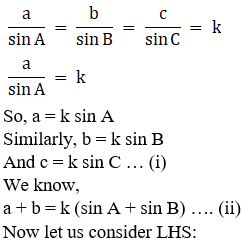• +91 9971497814
• info@interviewmaterial.com

# RD Chapter 10- Sine and Cosine Formulae and Their Applications Ex-10.1 Interview Questions Answers

### Related Subjects

Question 1 :

If in a∆ABC, A = 45oB = 60o,and C = 75o; findthe ratio of its sides.

Given: In ABC, A = 45oB = 60o,and C =75o

By using the sine rule, we get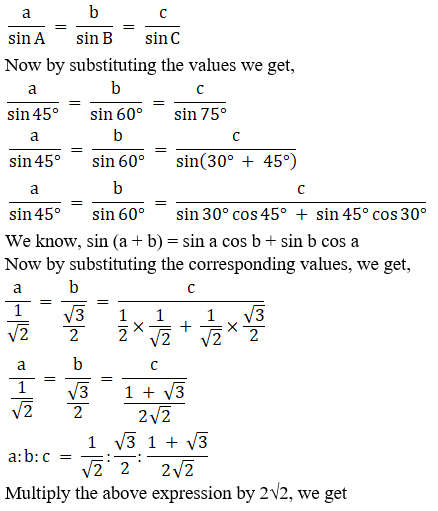a: b: c = 2: √6: (1+√3)

Hence the ratio of the sides of the given triangle isa: b: c = 2: √6: (1+√3)

Question 2 : If in any ∆ABC, ∠C = 105o, ∠B = 45o, a = 2, then find b.

Given: In ∆ABC, C = 105oB = 45o, a =2

We know in a triangle,

A + B + C = 180°

A = 180° – B – C

Substituting the given values, we get

A = 180° – 45° – 105°

A = 30°

By using the sine rule, we get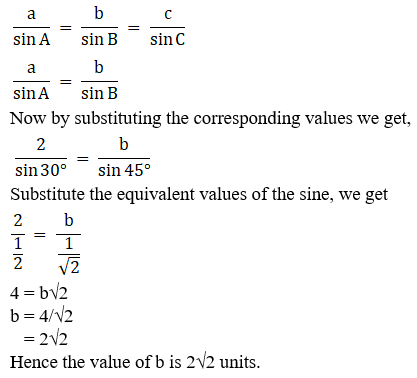Question 3 : In ∆ABC, if a = 18, b = 24 and c = 30 and ∠C = 90o, find sin A, sin B and sin C.

Given: In ∆ABC, a = 18, b = 24 and c = 30 and C = 90o

By using the sine rule, we get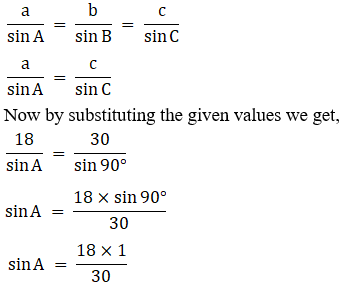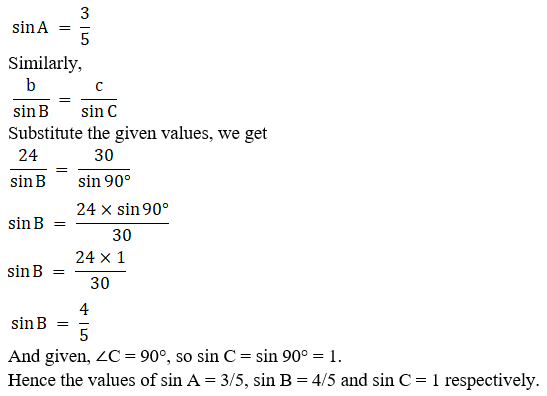In anytriangle ABC, prove the following:

Question 4 :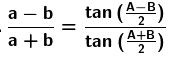By using the sine rule we know,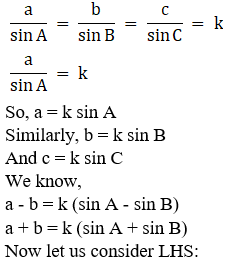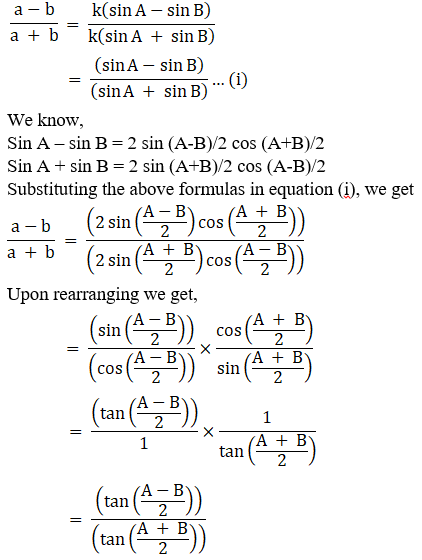= RHS

Hence proved.

Question 5 : (a – b) cos C/2 = C sin (A – B)/2

Answer 5 : By using the sine rule we know,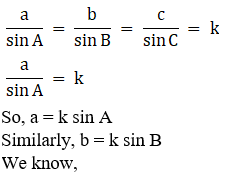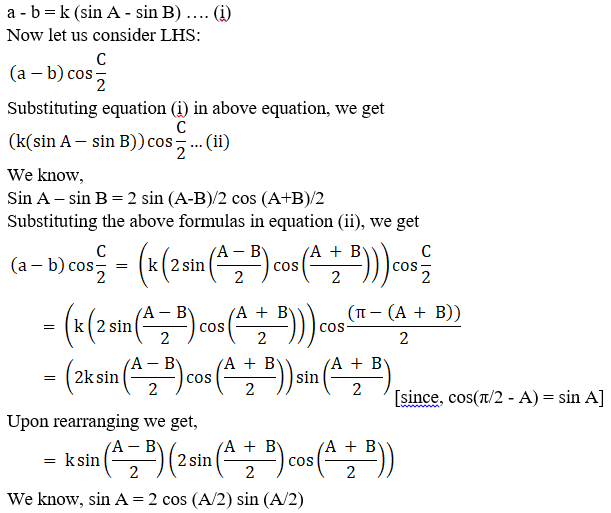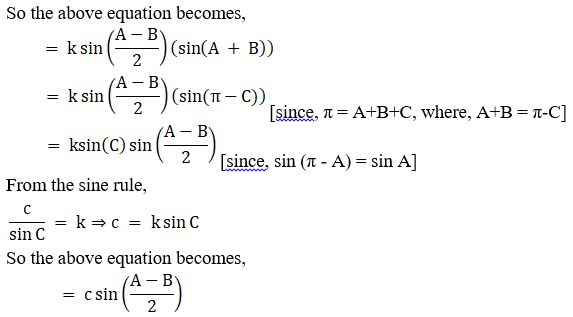= RHS

Hence proved.

Question 6 :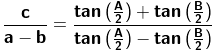Answer 6 : By using the sine rule we know,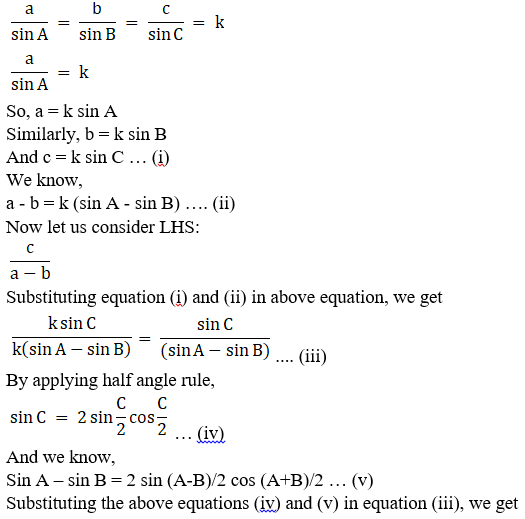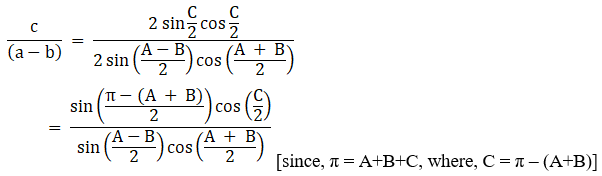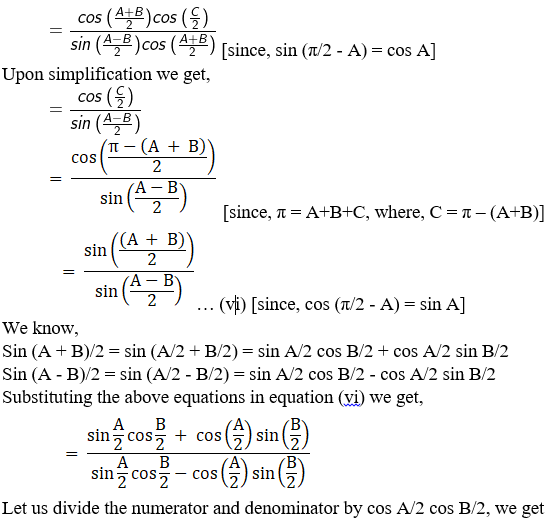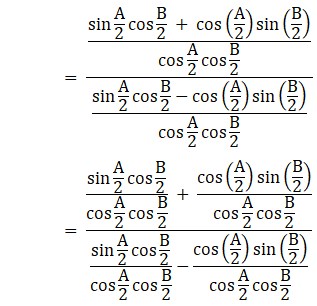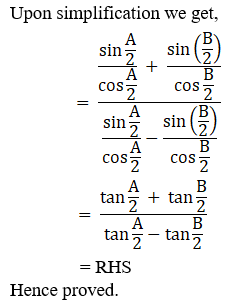Question 7 :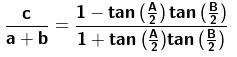Answer 7 : By using the sine rule we know,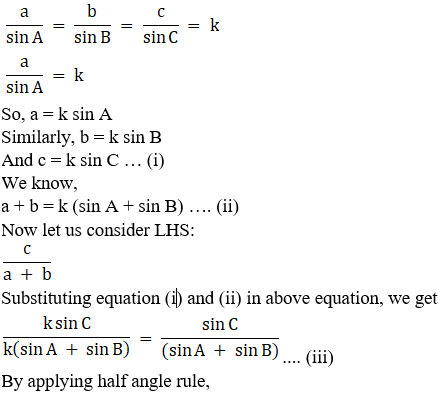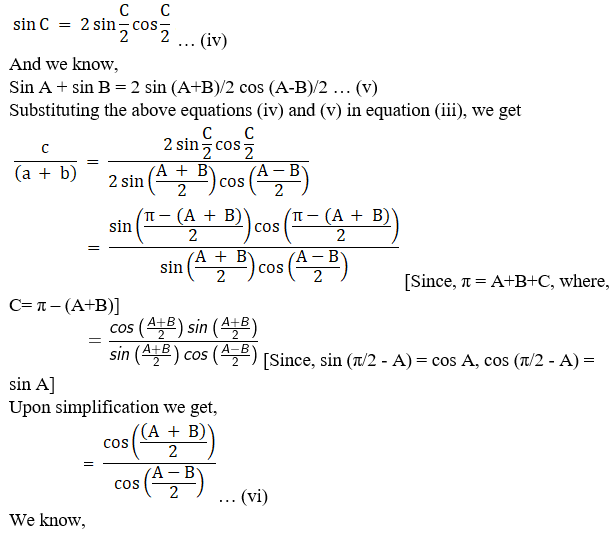cos (A + B)/2 = cos (A/2 + B/2) = cos A/2 cos B/2 +sin A/2 sin B/2

cos (A – B)/2 = cos (A/2 – B/2) = cos A/2 cos B/2 –sin A/2 sin B/2

Substituting the above equations in equation (vi) weget,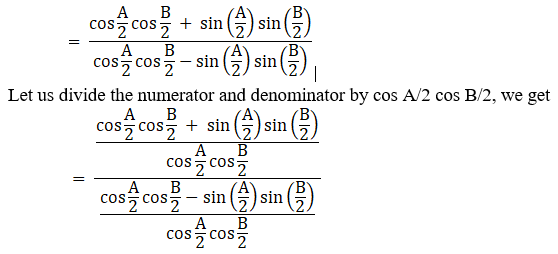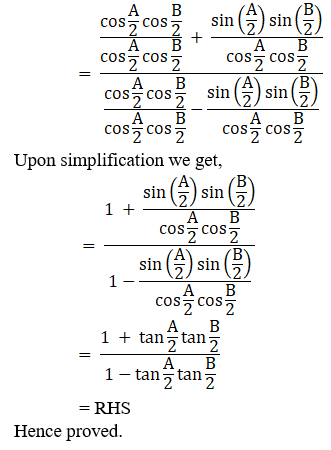Question 8 :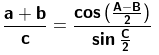Answer 8 : By using the sine rule we know,